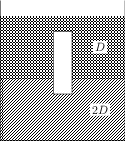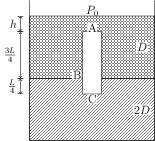# Buoyancy

## Problems from IIT JEE

Problem (IIT JEE 1995):A homogeneous solid cylinder of length $L$ and cross-sectional area $A/5$ is immersed such that it floats with its axis vertical at the liquid-liquid interface with length $L/4$ in the denser liquid as shown in the figure. The lower density liquid is open to atmosphere having pressure $P_0$. Then, density $D$ of solid is given by,

1. $\frac{5}{4}d$
2. $\frac{4}{5}d$
3. $4d$
4. $\frac{d}{5}$

Solution:Let A be the topmost point of the cylinder, B be the point on the interface, and C be the lowest point of the cylinder. Let the depth of point A be $h$. The pressures at A, B and C are, \begin{align} &P_A=P_0+hdg, \\ &P_B=P_A+\tfrac{3}{4}Ldg,\\ &P_C=P_B+\tfrac{L}{4}(2d)g\!=\!P_A+\tfrac{5}{4}Ldg. \end{align} The forces on the cylinder are its weight $W=D (LA/5) g$ (downwards), hydrostatic force at the top surface $F_A=P_A (A/5)$ (downwards), and hydrostatic force at the bottom surface $F_C=P_C (A/5)$ (upwards). In equilibrium, $F_A+W=F_C$ i.e., \begin{align} \label{bkb:eqn:4} P_A A/5+D (LA/5) g=(P_A+\tfrac{5}{4}Ldg) A/5. \end{align} Simplify above equation to get $D={5d}/{4}$.